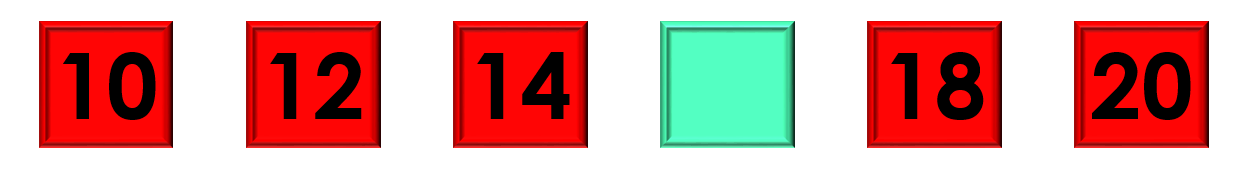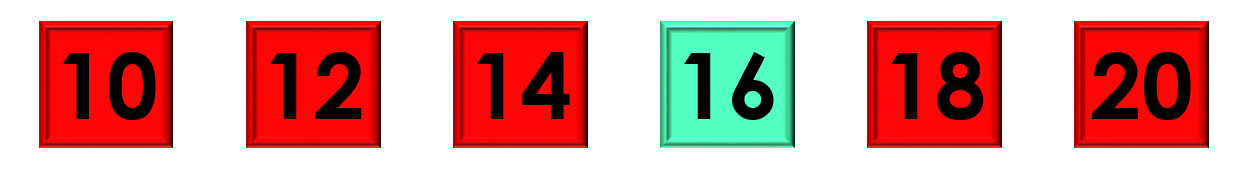1
visibility

Consider the given number pattern and identify the missing number.• A

13

• B

14

• C

15

• D

16

The correct answer is 16.

Solution:

Considering the given number pattern.Here, the numbers are increasing.
So, to find the missing number, we first find the rule.

Note that:
10 + 2 = 12
12 + 2 = 14
14 + 2 = 16
16 + 2 = 18
18 + 2 = 20

So, the rule is "add 2".Then, the missing number is 16.